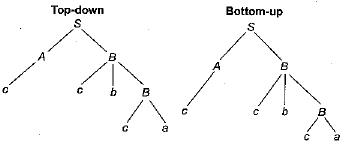Courses

# Parsing Techniques ( Advance Level ) - 1

## 15 Questions MCQ Test Question Bank for GATE Computer Science Engineering | Parsing Techniques ( Advance Level ) - 1

Description
This mock test of Parsing Techniques ( Advance Level ) - 1 for Computer Science Engineering (CSE) helps you for every Computer Science Engineering (CSE) entrance exam. This contains 15 Multiple Choice Questions for Computer Science Engineering (CSE) Parsing Techniques ( Advance Level ) - 1 (mcq) to study with solutions a complete question bank. The solved questions answers in this Parsing Techniques ( Advance Level ) - 1 quiz give you a good mix of easy questions and tough questions. Computer Science Engineering (CSE) students definitely take this Parsing Techniques ( Advance Level ) - 1 exercise for a better result in the exam. You can find other Parsing Techniques ( Advance Level ) - 1 extra questions, long questions & short questions for Computer Science Engineering (CSE) on EduRev as well by searching above.
QUESTION: 1

### Which of the following actions an operator- precedence parser may take to recover from an error?

Solution:

To recover from an error actions taken by operator precedence parser:
1. Insert symbols onto stack.
2. Delete symbols from the stack.
3. Insert or delete symbols from the input.

QUESTION: 2

### Which of the following is the important factor that justifies the use of a stack in shift-reduce parsing?

Solution:

In shift-reduce parsing "the handle will always appear inside the stack and reversor top”.

QUESTION: 3

### A shift reduce parser carries out the actions specified with in braces immediately after reducing the corresponding rule of grammar.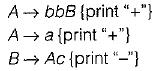What is the translation of bbbbacc using the syntax directed translation scheme described by the above rules?

Solution: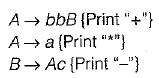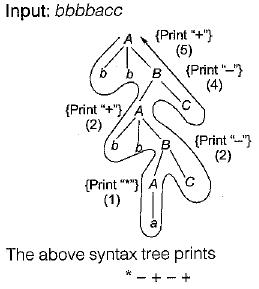QUESTION: 4

Which of the following statement is correct about parsing?
1. Top-down parsing expands the start symbol to the required string needed whereas in bottom-up parsing reduces the syntax to the start symbol.
2. Top-down parsing is implemented using the set of recursive procedures and implementation of bottom-up parsing is done using the stacks and input buffer.
3. Backtracking is required for both bottom-up and top-down parsing implementation.

Solution:
• Top down parsing expands the start symbol to the required string needed where as in bottom up parse string reduces to start symbol by handle purring.
• Top down parser also known as recursive descent parser i.e. uses recursive procedure, where as bottom up parser user stack and input buffer.
• Backbacking is required in top-down parser but not in bottom up.
QUESTION: 5

Which statement is true?

Solution:
• LALR is less powerful than CLR but more costly than any parser.
• Every SLR grammar is unambigous but every unambigous grammar need not be SLR i.e. may be LALR but not SLR.
• LR(K) grammar never using backtracking.
QUESTION: 6

For a context-free grammar, FOLLOW(A) is the set of terminals that can appear immediately to the right of non-terminal A in some “sentential” form. We defined to sets LFOLLOW(A) and RFOLLOW(A) by replacing the word “left most sentential” and “right most sentential” respectively in the definition of FOLLOW(A).

Which of the following statements is/are true

Solution:

Definition of Follow (A) as per question “The set of terminals that can appears immediately to the right of non-terminal A in same sentential form”. If the above definition is changed to replace “ sentential” with “ left-most sentential” for L FOLLOW (A) and “right-most sentential” for R FOLLOW (A)

We are still considering the terminal symbol to the right of A ’ .
Hence all three sets would be identical

QUESTION: 7

Consider the SLR(1) and LALR(1) parsing tables for a context free grammar. Which of the following statements is/are true?

Solution:

In SLR (1) and LALR (1) parsing tables for CFG shift entries and Goto part are identical but reduced entries and error entries may be different

QUESTION: 8

Which of the following statements is false?

Solution:

An ambiguous grammar have more than one leftmost or rightmost derivation tree.

QUESTION: 9

Consider the following expression grammar, The semantic rules for expression calculation-are stated next to each grammar production.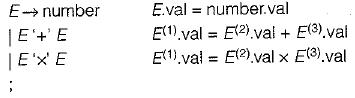The above grammar and the semantic rules are fed to a yacc tool (which is an LALR(1) parser generator) for parsing and evaluating arithmetic expressions. Which one of the following is true about the action of yacc for the given grammar?

Solution:

E→ number \ E' + E' \ E' x E'

Then YACC compiler detects shift-reduce conflict, and resolves the conflict in favor of a shift over a reduce action.
Consider the following configuration where shift- reduce conflict occurs

Stack Input

.... E + E x . E . . \$

QUESTION: 10

Which of the following describes a handle (as applicable to LR-parsing) appropriately?

Solution:

A handle may be described as the sentencial form given by the bottom up parser from left to right in reverse order.

QUESTION: 11

An LALR(1) parser for a grammar G can have shift-reduce (S-R) conflicts if and only if

Solution:

LALR(1) parser for a grammar, G can have shift- reduce ( S - R) conflicts if and only if there is a S - R conflict in its immediate sub-parsers (e.g. LR(1)). If LR(0) has S - R conflicts then they may get removed in LR (1), hence there will be no S - R conflict in LALR(1).

QUESTION: 12

Consider the following grammar production: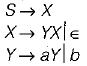Which of the following is not LR(1) item set?

Solution: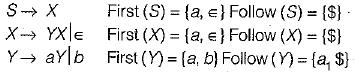So, parsing table must not contain entry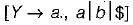since Y→a production not present in grammar.

QUESTION: 13

Consider the production:

S→ aS | b

The parsing automation is below: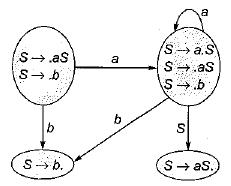Which of the following stack contents causes the parser to reduce by some production?

Solution: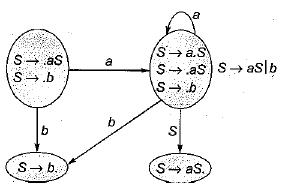Some possible stack contents are aaS, ab, b, etc.

QUESTION: 14

Consider the following grammar production: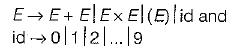The expression 3 + 2 x 5 is parsed by the shift- reduce parser then which of the following is not a handle for it?

Solution: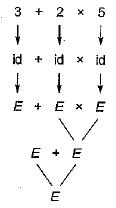So E + E x E is not a handle for the expression.

QUESTION: 15

Consider a grammar: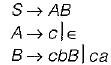The input string ccbca is parsed by which of the following parsing method?

Solution:

Given grammar can be parsed using both Top- down and bottom up parsing methods for the input string “ccbca"